Algebra II : Negative Exponents

Example Questions

← Previous 1 3 4 5 6 7 8 9 10 11 12

Example Question #1 : Negative Exponents

Simplify the following expression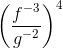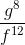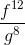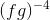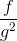Explanation: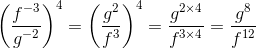Example Question #2 : Exponents

Simplify the following expression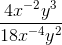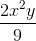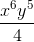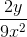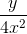Explanation: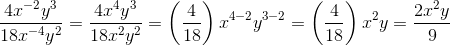Example Question #3 : Exponents

Simplify the following expression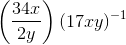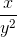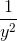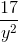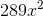Explanation: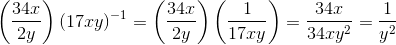Example Question #4 : Exponents

Simplify the following expression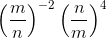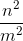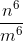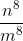Explanation: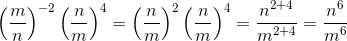Example Question #5 : Exponents

Simplify the following expression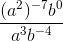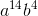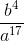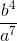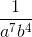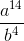Explanation: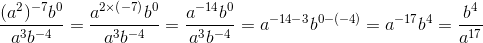Example Question #1 : Negative Exponents

Solve for: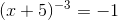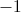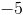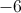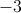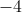Explanation:

Raise both sides of the equation to the inverse power ofto cancel the exponent on the left hand side of the equation.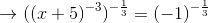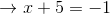Subtractfrom both sides: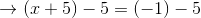Example Question #7 : Exponents

Represent the fraction using only positive exponents: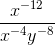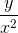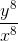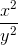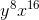Explanation:Negative exponents are the reciprocal of their positive counterpart. For example: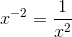Therefore: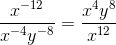This simplifies to:Example Question #8 : Exponents

Solve the equation for n: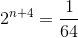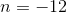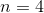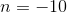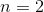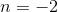Explanation:

Rewrite the right-hand-side so that each side has the same base: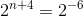Use the Property of Equality for Exponential Functions: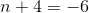Solving for:Example Question #9 : Exponents

What is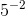the same as?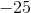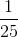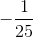Explanation:

While a positive exponent says how many times to multiply by a number, a negative exponent says how many times to divide by the number.

To solve for negative exponents, just calculate the reciprocal.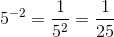Example Question #10 : Exponents

Solve: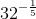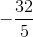Explanation:

To evaluate a negative exponent, convert the exponent to positive by taking the inverse.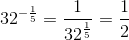← Previous 1 3 4 5 6 7 8 9 10 11 12

All Algebra II Resources